Anúncio

# Heat transfer

Assistant Professor, Dr.Shivajirao Kadam College of Pharmacy,Kasabe Digraj,Sangli.
16 de Aug de 2019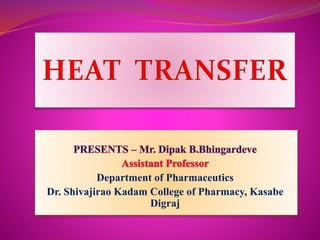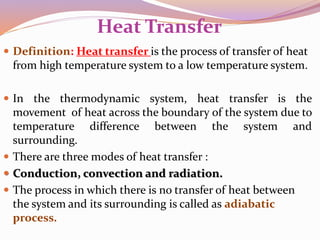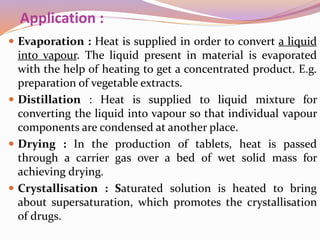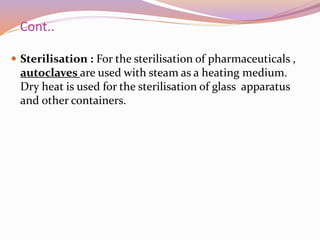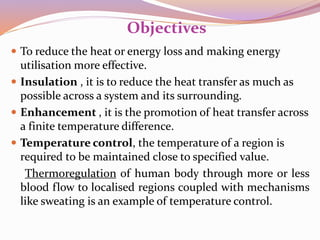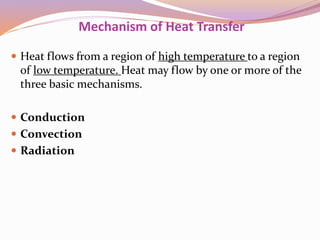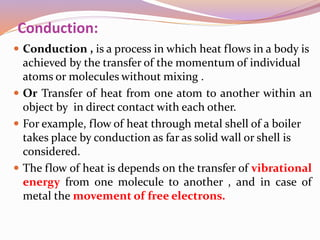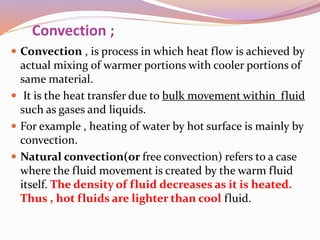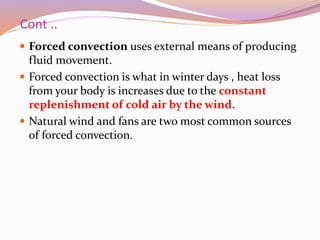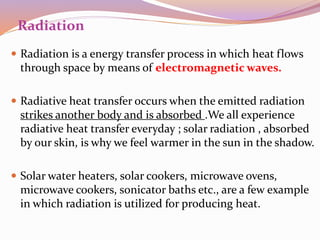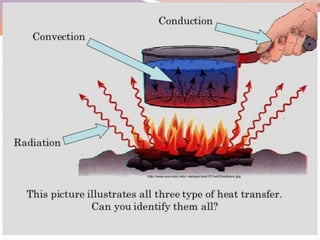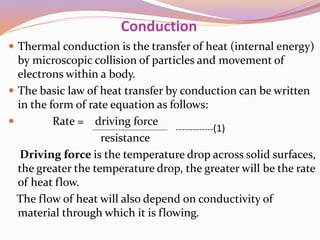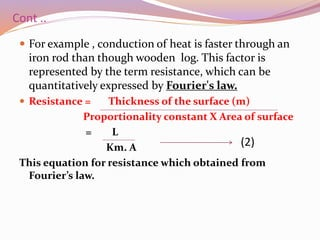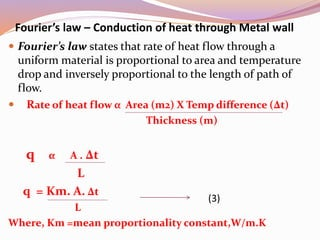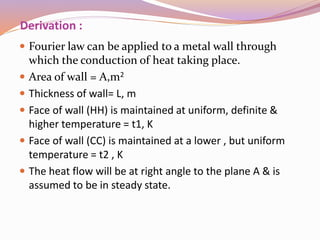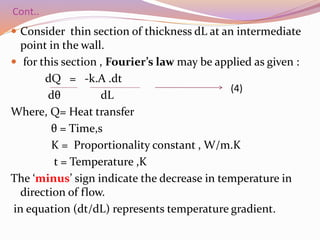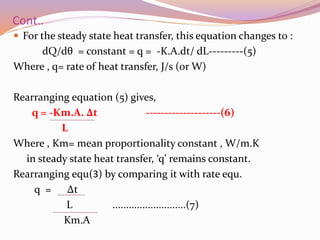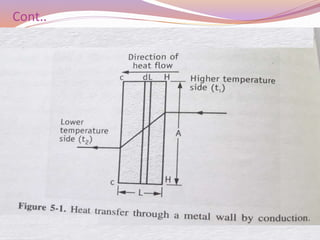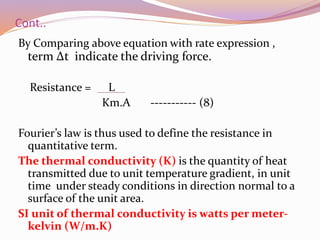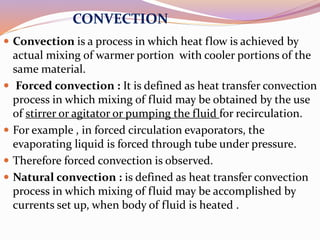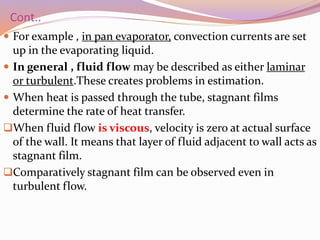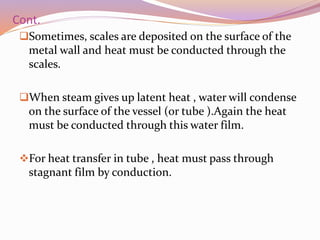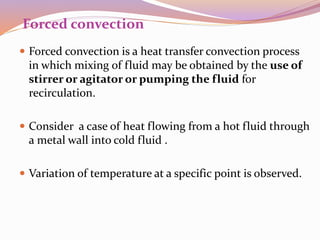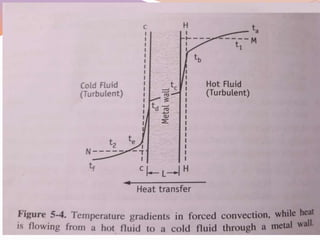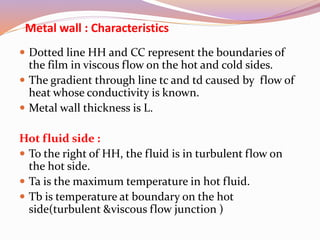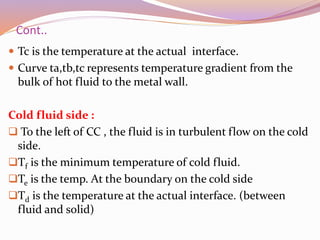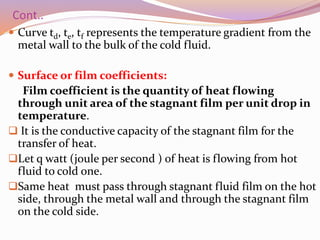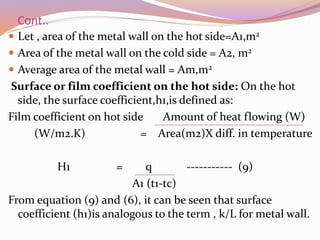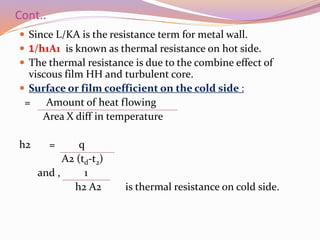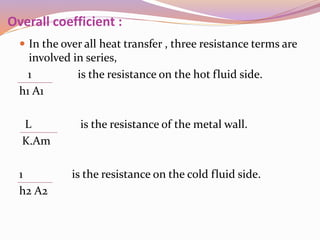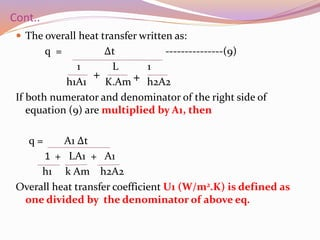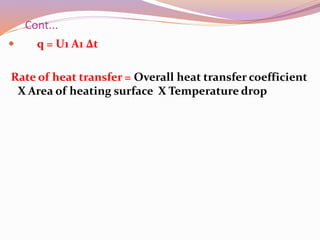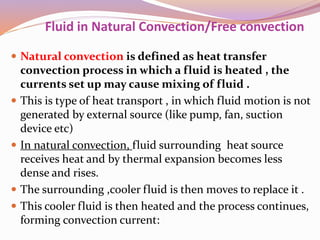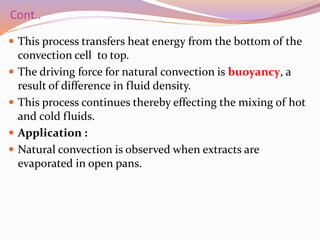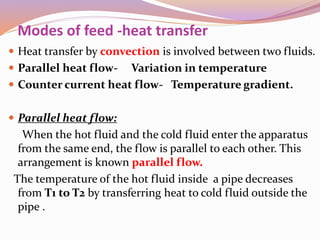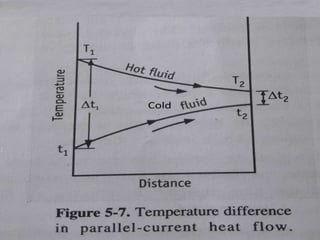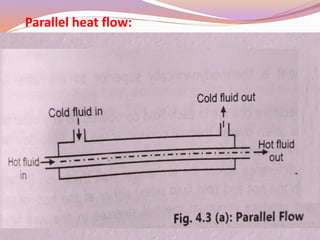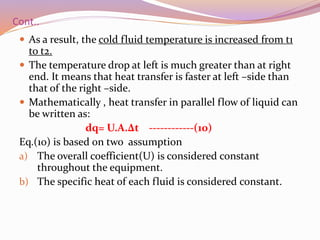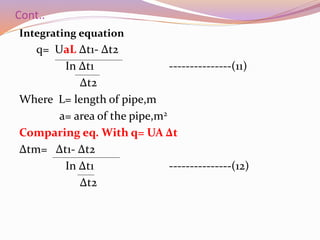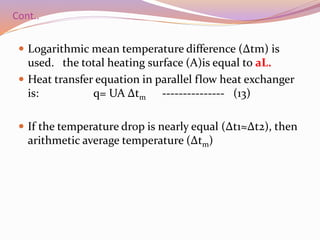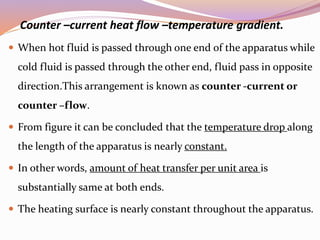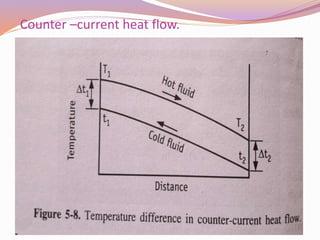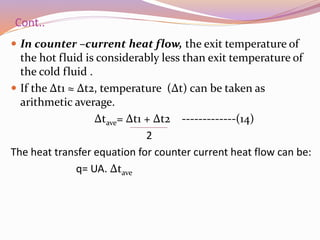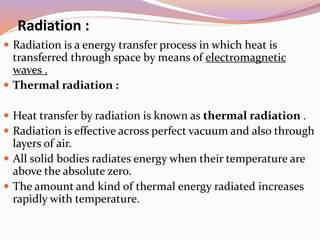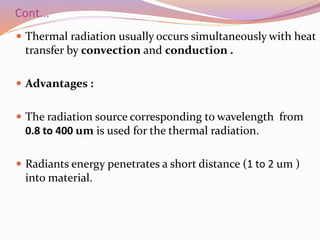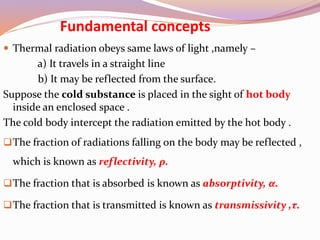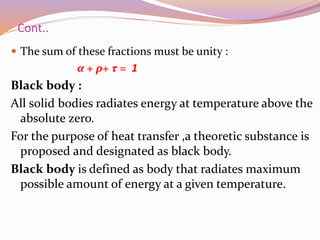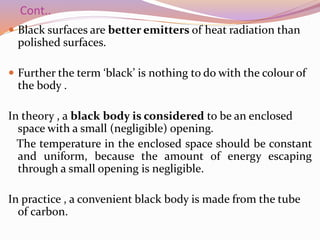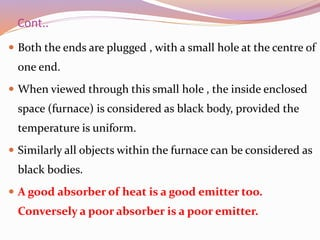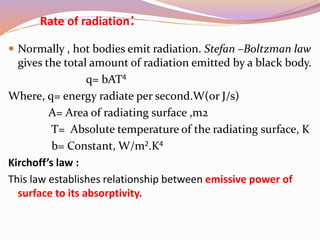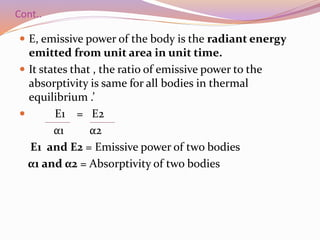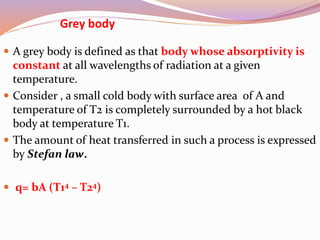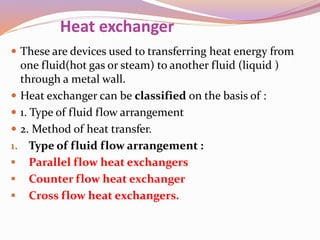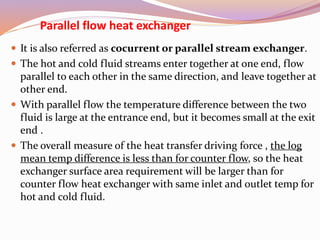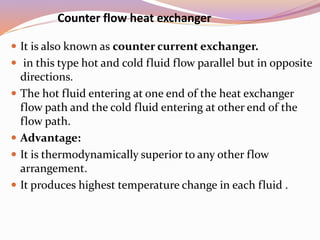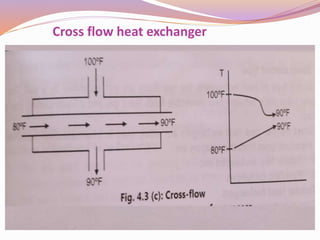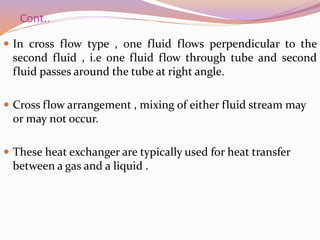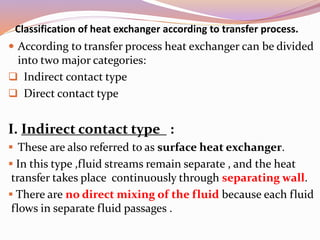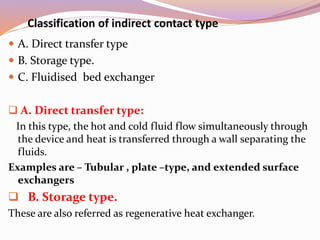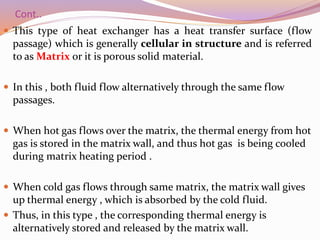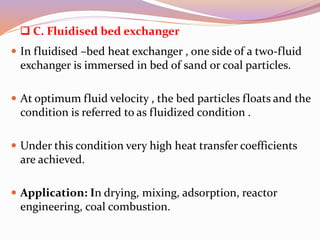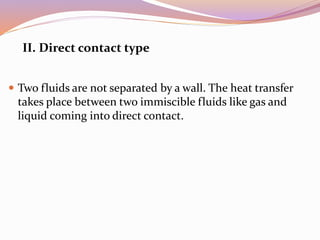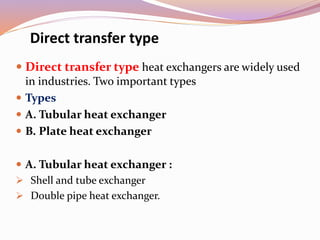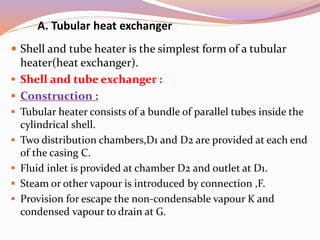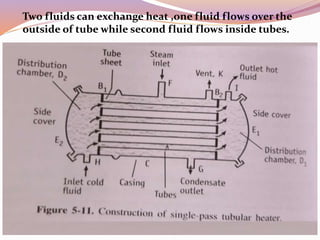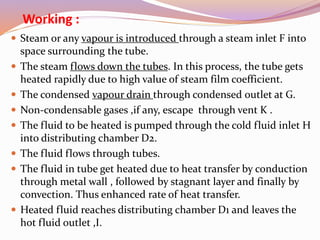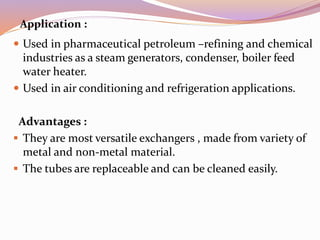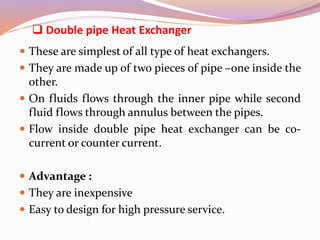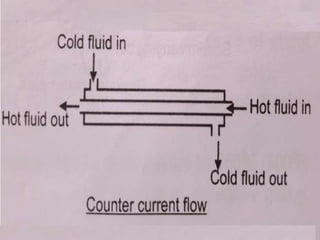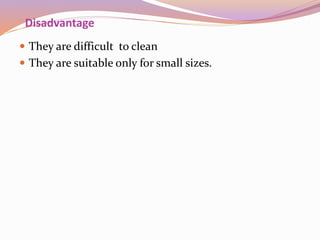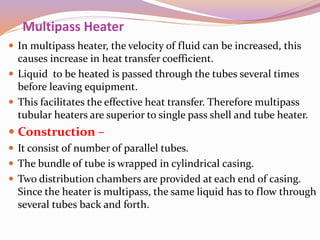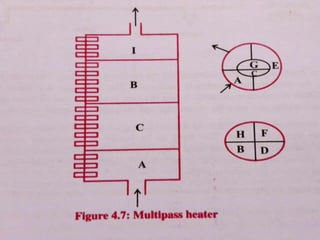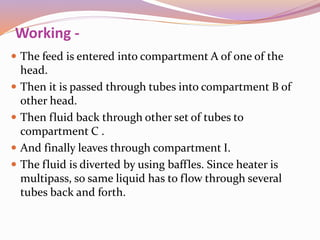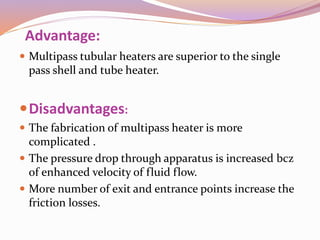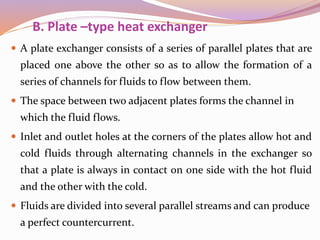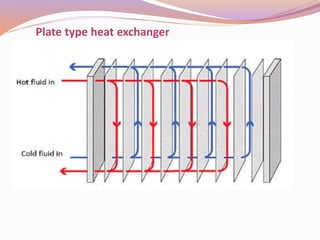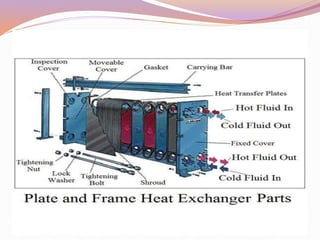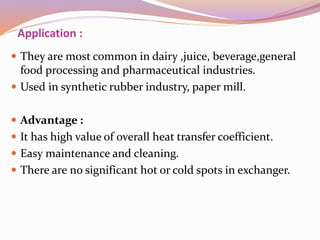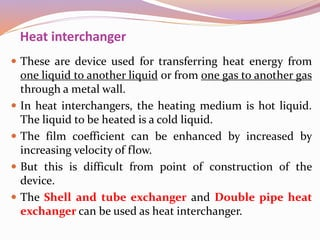1 de 82
Anúncio

### Heat transfer

1. Department of Pharmaceutics Dr. Shivajirao Kadam College of Pharmacy, Kasabe Digraj
2. Heat Transfer  Definition: Heat transfer is the process of transfer of heat from high temperature system to a low temperature system.  In the thermodynamic system, heat transfer is the movement of heat across the boundary of the system due to temperature difference between the system and surrounding.  There are three modes of heat transfer :  Conduction, convection and radiation.  The process in which there is no transfer of heat between the system and its surrounding is called as adiabatic process.
3. Application :  Evaporation : Heat is supplied in order to convert a liquid into vapour. The liquid present in material is evaporated with the help of heating to get a concentrated product. E.g. preparation of vegetable extracts.  Distillation : Heat is supplied to liquid mixture for converting the liquid into vapour so that individual vapour components are condensed at another place.  Drying : In the production of tablets, heat is passed through a carrier gas over a bed of wet solid mass for achieving drying.  Crystallisation : Saturated solution is heated to bring about supersaturation, which promotes the crystallisation of drugs.
4. Cont..  Sterilisation : For the sterilisation of pharmaceuticals , autoclaves are used with steam as a heating medium. Dry heat is used for the sterilisation of glass apparatus and other containers.
5. Objectives  To reduce the heat or energy loss and making energy utilisation more effective.  Insulation , it is to reduce the heat transfer as much as possible across a system and its surrounding.  Enhancement , it is the promotion of heat transfer across a finite temperature difference.  Temperature control, the temperature of a region is required to be maintained close to specified value. Thermoregulation of human body through more or less blood flow to localised regions coupled with mechanisms like sweating is an example of temperature control.
6. Mechanism of Heat Transfer  Heat flows from a region of high temperature to a region of low temperature. Heat may flow by one or more of the three basic mechanisms.  Conduction  Convection  Radiation
7. Conduction:  Conduction , is a process in which heat flows in a body is achieved by the transfer of the momentum of individual atoms or molecules without mixing .  Or Transfer of heat from one atom to another within an object by in direct contact with each other.  For example, flow of heat through metal shell of a boiler takes place by conduction as far as solid wall or shell is considered.  The flow of heat is depends on the transfer of vibrational energy from one molecule to another , and in case of metal the movement of free electrons.
8. Convection ;  Convection , is process in which heat flow is achieved by actual mixing of warmer portions with cooler portions of same material.  It is the heat transfer due to bulk movement within fluid such as gases and liquids.  For example , heating of water by hot surface is mainly by convection.  Natural convection(or free convection) refers to a case where the fluid movement is created by the warm fluid itself. The density of fluid decreases as it is heated. Thus , hot fluids are lighter than cool fluid.
9. Cont ..  Forced convection uses external means of producing fluid movement.  Forced convection is what in winter days , heat loss from your body is increases due to the constant replenishment of cold air by the wind.  Natural wind and fans are two most common sources of forced convection.
10. Radiation  Radiation is a energy transfer process in which heat flows through space by means of electromagnetic waves.  Radiative heat transfer occurs when the emitted radiation strikes another body and is absorbed .We all experience radiative heat transfer everyday ; solar radiation , absorbed by our skin, is why we feel warmer in the sun in the shadow.  Solar water heaters, solar cookers, microwave ovens, microwave cookers, sonicator baths etc., are a few example in which radiation is utilized for producing heat.
11. Conduction  Thermal conduction is the transfer of heat (internal energy) by microscopic collision of particles and movement of electrons within a body.  The basic law of heat transfer by conduction can be written in the form of rate equation as follows:  Rate = driving force resistance Driving force is the temperature drop across solid surfaces, the greater the temperature drop, the greater will be the rate of heat flow. The flow of heat will also depend on conductivity of material through which it is flowing. -------------(1)
12. Cont ..  For example , conduction of heat is faster through an iron rod than though wooden log. This factor is represented by the term resistance, which can be quantitatively expressed by Fourier's law.  Resistance = Thickness of the surface (m) Proportionality constant Χ Area of surface = L Km. A This equation for resistance which obtained from Fourier’s law. (2)
13. Fourier’s law – Conduction of heat through Metal wall  Fourier’s law states that rate of heat flow through a uniform material is proportional to area and temperature drop and inversely proportional to the length of path of flow.  Rate of heat flow α Area (m2) Χ Temp difference (Δt) Thickness (m) q α A . Δt L q = Km. A. Δt L Where, Km =mean proportionality constant,W/m.K (3)
14. Derivation :  Fourier law can be applied to a metal wall through which the conduction of heat taking place.  Area of wall = A,m2  Thickness of wall= L, m  Face of wall (HH) is maintained at uniform, definite & higher temperature = t1, K  Face of wall (CC) is maintained at a lower , but uniform temperature = t2 , K  The heat flow will be at right angle to the plane A & is assumed to be in steady state.
15. Cont..  Consider thin section of thickness dL at an intermediate point in the wall.  for this section , Fourier’s law may be applied as given : dQ = -k.A .dt dθ dL Where, Q= Heat transfer θ = Time,s K = Proportionality constant , W/m.K t = Temperature ,K The ‘minus’ sign indicate the decrease in temperature in direction of flow. in equation (dt/dL) represents temperature gradient. (4)
16. Cont..  For the steady state heat transfer, this equation changes to : dQ/dθ = constant = q = -K.A.dt/ dL---------(5) Where , q= rate of heat transfer, J/s (or W) Rearranging equation (5) gives, q = -Km.A. Δt --------------------(6) L Where , Km= mean proportionality constant , W/m.K in steady state heat transfer, ‘q’ remains constant. Rearranging equ(3) by comparing it with rate equ. q = Δt L ...........................(7) Km.A
17. Cont..
18. Cont.. By Comparing above equation with rate expression , term Δt indicate the driving force. Resistance = L Km.A ----------- (8) Fourier’s law is thus used to define the resistance in quantitative term. The thermal conductivity (K) is the quantity of heat transmitted due to unit temperature gradient, in unit time under steady conditions in direction normal to a surface of the unit area. SI unit of thermal conductivity is watts per meter- kelvin (W/m.K)
19. CONVECTION  Convection is a process in which heat flow is achieved by actual mixing of warmer portion with cooler portions of the same material.  Forced convection : It is defined as heat transfer convection process in which mixing of fluid may be obtained by the use of stirrer or agitator or pumping the fluid for recirculation.  For example , in forced circulation evaporators, the evaporating liquid is forced through tube under pressure.  Therefore forced convection is observed.  Natural convection : is defined as heat transfer convection process in which mixing of fluid may be accomplished by currents set up, when body of fluid is heated .
20. Cont..  For example , in pan evaporator, convection currents are set up in the evaporating liquid.  In general , fluid flow may be described as either laminar or turbulent.These creates problems in estimation.  When heat is passed through the tube, stagnant films determine the rate of heat transfer. When fluid flow is viscous, velocity is zero at actual surface of the wall. It means that layer of fluid adjacent to wall acts as stagnant film. Comparatively stagnant film can be observed even in turbulent flow.
21. Cont. Sometimes, scales are deposited on the surface of the metal wall and heat must be conducted through the scales. When steam gives up latent heat , water will condense on the surface of the vessel (or tube ).Again the heat must be conducted through this water film. For heat transfer in tube , heat must pass through stagnant film by conduction.
22. Forced convection  Forced convection is a heat transfer convection process in which mixing of fluid may be obtained by the use of stirrer or agitator or pumping the fluid for recirculation.  Consider a case of heat flowing from a hot fluid through a metal wall into cold fluid .  Variation of temperature at a specific point is observed.
23. Metal wall : Characteristics  Dotted line HH and CC represent the boundaries of the film in viscous flow on the hot and cold sides.  The gradient through line tc and td caused by flow of heat whose conductivity is known.  Metal wall thickness is L. Hot fluid side :  To the right of HH, the fluid is in turbulent flow on the hot side.  Ta is the maximum temperature in hot fluid.  Tb is temperature at boundary on the hot side(turbulent &viscous flow junction )
24. Cont..  Tc is the temperature at the actual interface.  Curve ta,tb,tc represents temperature gradient from the bulk of hot fluid to the metal wall. Cold fluid side :  To the left of CC , the fluid is in turbulent flow on the cold side. Tf is the minimum temperature of cold fluid. Te is the temp. At the boundary on the cold side Td is the temperature at the actual interface. (between fluid and solid)
25. Cont..  Curve td, te, tf represents the temperature gradient from the metal wall to the bulk of the cold fluid.  Surface or film coefficients: Film coefficient is the quantity of heat flowing through unit area of the stagnant film per unit drop in temperature.  It is the conductive capacity of the stagnant film for the transfer of heat. Let q watt (joule per second ) of heat is flowing from hot fluid to cold one. Same heat must pass through stagnant fluid film on the hot side, through the metal wall and through the stagnant film on the cold side.
26. Cont..  Let , area of the metal wall on the hot side=A1,m2  Area of the metal wall on the cold side = A2, m2  Average area of the metal wall = Am,m2 Surface or film coefficient on the hot side: On the hot side, the surface coefficient,h1,is defined as: Film coefficient on hot side Amount of heat flowing (W) (W/m2.K) = Area(m2)Χ diff. in temperature H1 = q ----------- (9) A1 (t1-tc) From equation (9) and (6), it can be seen that surface coefficient (h1)is analogous to the term , k/L for metal wall.
27. Cont..  Since L/KA is the resistance term for metal wall.  1/h1A1 is known as thermal resistance on hot side.  The thermal resistance is due to the combine effect of viscous film HH and turbulent core.  Surface or film coefficient on the cold side : = Amount of heat flowing Area Χ diff in temperature h2 = q A2 (td-t2) and , 1 h2 A2 is thermal resistance on cold side.
28. Overall coefficient :  In the over all heat transfer , three resistance terms are involved in series, 1 is the resistance on the hot fluid side. h1 A1 L is the resistance of the metal wall. K.Am 1 is the resistance on the cold fluid side. h2 A2
29. Cont..  The overall heat transfer written as: q = Δt ---------------(9) 1 L 1 h1A1 K.Am h2A2 If both numerator and denominator of the right side of equation (9) are multiplied by A1, then q = A1 Δt 1 + LA1 + A1 h1 k Am h2A2 Overall heat transfer coefficient U1 (W/m2.K) is defined as one divided by the denominator of above eq. + +
30. Cont...  q = U1 A1 Δt Rate of heat transfer = Overall heat transfer coefficient Χ Area of heating surface Χ Temperature drop
31. Fluid in Natural Convection/Free convection  Natural convection is defined as heat transfer convection process in which a fluid is heated , the currents set up may cause mixing of fluid .  This is type of heat transport , in which fluid motion is not generated by external source (like pump, fan, suction device etc)  In natural convection, fluid surrounding heat source receives heat and by thermal expansion becomes less dense and rises.  The surrounding ,cooler fluid is then moves to replace it .  This cooler fluid is then heated and the process continues, forming convection current:
32. Cont..  This process transfers heat energy from the bottom of the convection cell to top.  The driving force for natural convection is buoyancy, a result of difference in fluid density.  This process continues thereby effecting the mixing of hot and cold fluids.  Application :  Natural convection is observed when extracts are evaporated in open pans.
33. Modes of feed -heat transfer  Heat transfer by convection is involved between two fluids.  Parallel heat flow- Variation in temperature  Counter current heat flow- Temperature gradient.  Parallel heat flow: When the hot fluid and the cold fluid enter the apparatus from the same end, the flow is parallel to each other. This arrangement is known parallel flow. The temperature of the hot fluid inside a pipe decreases from T1 to T2 by transferring heat to cold fluid outside the pipe .
34. cont..
35. Parallel heat flow:
36. Cont..  As a result, the cold fluid temperature is increased from t1 to t2.  The temperature drop at left is much greater than at right end. It means that heat transfer is faster at left –side than that of the right –side.  Mathematically , heat transfer in parallel flow of liquid can be written as: dq= U.A.Δt ------------(10) Eq.(10) is based on two assumption a) The overall coefficient(U) is considered constant throughout the equipment. b) The specific heat of each fluid is considered constant.
37. Cont.. Integrating equation q= UaL Δt1- Δt2 In Δt1 ---------------(11) Δt2 Where L= length of pipe,m a= area of the pipe,m2 Comparing eq. With q= UA Δt Δtm= Δt1- Δt2 In Δt1 ---------------(12) Δt2
38. Cont..  Logarithmic mean temperature difference (Δtm) is used. the total heating surface (A)is equal to aL.  Heat transfer equation in parallel flow heat exchanger is: q= UA Δtm --------------- (13)  If the temperature drop is nearly equal (Δt1≈Δt2), then arithmetic average temperature (Δtm)
39. Counter –current heat flow –temperature gradient.  When hot fluid is passed through one end of the apparatus while cold fluid is passed through the other end, fluid pass in opposite direction.This arrangement is known as counter -current or counter –flow.  From figure it can be concluded that the temperature drop along the length of the apparatus is nearly constant.  In other words, amount of heat transfer per unit area is substantially same at both ends.  The heating surface is nearly constant throughout the apparatus.
40. Counter –current heat flow.
41. Cont..  In counter –current heat flow, the exit temperature of the hot fluid is considerably less than exit temperature of the cold fluid .  If the Δt1 ≈ Δt2, temperature (Δt) can be taken as arithmetic average. Δtave= Δt1 + Δt2 -------------(14) 2 The heat transfer equation for counter current heat flow can be: q= UA. Δtave
42. Radiation :  Radiation is a energy transfer process in which heat is transferred through space by means of electromagnetic waves .  Thermal radiation :  Heat transfer by radiation is known as thermal radiation .  Radiation is effective across perfect vacuum and also through layers of air.  All solid bodies radiates energy when their temperature are above the absolute zero.  The amount and kind of thermal energy radiated increases rapidly with temperature.
43. Cont...  Thermal radiation usually occurs simultaneously with heat transfer by convection and conduction .  Advantages :  The radiation source corresponding to wavelength from 0.8 to 400 um is used for the thermal radiation.  Radiants energy penetrates a short distance (1 to 2 um ) into material.
44. Fundamental concepts  Thermal radiation obeys same laws of light ,namely – a) It travels in a straight line b) It may be reflected from the surface. Suppose the cold substance is placed in the sight of hot body inside an enclosed space . The cold body intercept the radiation emitted by the hot body . The fraction of radiations falling on the body may be reflected , which is known as reflectivity, ρ. The fraction that is absorbed is known as absorptivity, α. The fraction that is transmitted is known as transmissivity ,τ.
45. Cont..  The sum of these fractions must be unity : α + ρ+ τ = 1 Black body : All solid bodies radiates energy at temperature above the absolute zero. For the purpose of heat transfer ,a theoretic substance is proposed and designated as black body. Black body is defined as body that radiates maximum possible amount of energy at a given temperature.
46. Cont..  Black surfaces are better emitters of heat radiation than polished surfaces.  Further the term ‘black’ is nothing to do with the colour of the body . In theory , a black body is considered to be an enclosed space with a small (negligible) opening. The temperature in the enclosed space should be constant and uniform, because the amount of energy escaping through a small opening is negligible. In practice , a convenient black body is made from the tube of carbon.
47. Cont..  Both the ends are plugged , with a small hole at the centre of one end.  When viewed through this small hole , the inside enclosed space (furnace) is considered as black body, provided the temperature is uniform.  Similarly all objects within the furnace can be considered as black bodies.  A good absorber of heat is a good emitter too. Conversely a poor absorber is a poor emitter.
48. Rate of radiation:  Normally , hot bodies emit radiation. Stefan –Boltzman law gives the total amount of radiation emitted by a black body. q= bAT4 Where, q= energy radiate per second.W(or J/s) A= Area of radiating surface ,m2 T= Absolute temperature of the radiating surface, K b= Constant, W/m2.K4 Kirchoff’s law : This law establishes relationship between emissive power of surface to its absorptivity.
49. Cont..  E, emissive power of the body is the radiant energy emitted from unit area in unit time.  It states that , the ratio of emissive power to the absorptivity is same for all bodies in thermal equilibrium .’  E1 = E2 α1 α2 E1 and E2 = Emissive power of two bodies α1 and α2 = Absorptivity of two bodies
50. Grey body  A grey body is defined as that body whose absorptivity is constant at all wavelengths of radiation at a given temperature.  Consider , a small cold body with surface area of A and temperature of T2 is completely surrounded by a hot black body at temperature T1.  The amount of heat transferred in such a process is expressed by Stefan law.  q= bA (T14 – T24)
51. Heat exchanger  These are devices used to transferring heat energy from one fluid(hot gas or steam) to another fluid (liquid ) through a metal wall.  Heat exchanger can be classified on the basis of :  1. Type of fluid flow arrangement  2. Method of heat transfer. 1. Type of fluid flow arrangement :  Parallel flow heat exchangers  Counter flow heat exchanger  Cross flow heat exchangers.
52. Parallel flow heat exchanger  It is also referred as cocurrent or parallel stream exchanger.  The hot and cold fluid streams enter together at one end, flow parallel to each other in the same direction, and leave together at other end.  With parallel flow the temperature difference between the two fluid is large at the entrance end, but it becomes small at the exit end .  The overall measure of the heat transfer driving force , the log mean temp difference is less than for counter flow, so the heat exchanger surface area requirement will be larger than for counter flow heat exchanger with same inlet and outlet temp for hot and cold fluid.
53. Counter flow heat exchanger  It is also known as counter current exchanger.  in this type hot and cold fluid flow parallel but in opposite directions.  The hot fluid entering at one end of the heat exchanger flow path and the cold fluid entering at other end of the flow path.  Advantage:  It is thermodynamically superior to any other flow arrangement.  It produces highest temperature change in each fluid .
54. Cross flow heat exchanger
55. Cont..  In cross flow type , one fluid flows perpendicular to the second fluid , i.e one fluid flow through tube and second fluid passes around the tube at right angle.  Cross flow arrangement , mixing of either fluid stream may or may not occur.  These heat exchanger are typically used for heat transfer between a gas and a liquid .
56. Classification of heat exchanger according to transfer process.  According to transfer process heat exchanger can be divided into two major categories:  Indirect contact type  Direct contact type I. Indirect contact type :  These are also referred to as surface heat exchanger.  In this type ,fluid streams remain separate , and the heat transfer takes place continuously through separating wall.  There are no direct mixing of the fluid because each fluid flows in separate fluid passages .
57. Classification of indirect contact type  A. Direct transfer type  B. Storage type.  C. Fluidised bed exchanger  A. Direct transfer type: In this type, the hot and cold fluid flow simultaneously through the device and heat is transferred through a wall separating the fluids. Examples are – Tubular , plate –type, and extended surface exchangers  B. Storage type. These are also referred as regenerative heat exchanger.
58. Cont..  This type of heat exchanger has a heat transfer surface (flow passage) which is generally cellular in structure and is referred to as Matrix or it is porous solid material.  In this , both fluid flow alternatively through the same flow passages.  When hot gas flows over the matrix, the thermal energy from hot gas is stored in the matrix wall, and thus hot gas is being cooled during matrix heating period .  When cold gas flows through same matrix, the matrix wall gives up thermal energy , which is absorbed by the cold fluid.  Thus, in this type , the corresponding thermal energy is alternatively stored and released by the matrix wall.
59.  C. Fluidised bed exchanger  In fluidised –bed heat exchanger , one side of a two-fluid exchanger is immersed in bed of sand or coal particles.  At optimum fluid velocity , the bed particles floats and the condition is referred to as fluidized condition .  Under this condition very high heat transfer coefficients are achieved.  Application: In drying, mixing, adsorption, reactor engineering, coal combustion.
60. II. Direct contact type  Two fluids are not separated by a wall. The heat transfer takes place between two immiscible fluids like gas and liquid coming into direct contact.
61. Direct transfer type  Direct transfer type heat exchangers are widely used in industries. Two important types  Types  A. Tubular heat exchanger  B. Plate heat exchanger  A. Tubular heat exchanger :  Shell and tube exchanger  Double pipe heat exchanger.
62. A. Tubular heat exchanger  Shell and tube heater is the simplest form of a tubular heater(heat exchanger).  Shell and tube exchanger :  Construction :  Tubular heater consists of a bundle of parallel tubes inside the cylindrical shell.  Two distribution chambers,D1 and D2 are provided at each end of the casing C.  Fluid inlet is provided at chamber D2 and outlet at D1.  Steam or other vapour is introduced by connection ,F.  Provision for escape the non-condensable vapour K and condensed vapour to drain at G.
63. Two fluids can exchange heat ,one fluid flows over the outside of tube while second fluid flows inside tubes.
64. Working :  Steam or any vapour is introduced through a steam inlet F into space surrounding the tube.  The steam flows down the tubes. In this process, the tube gets heated rapidly due to high value of steam film coefficient.  The condensed vapour drain through condensed outlet at G.  Non-condensable gases ,if any, escape through vent K .  The fluid to be heated is pumped through the cold fluid inlet H into distributing chamber D2.  The fluid flows through tubes.  The fluid in tube get heated due to heat transfer by conduction through metal wall , followed by stagnant layer and finally by convection. Thus enhanced rate of heat transfer.  Heated fluid reaches distributing chamber D1 and leaves the hot fluid outlet ,I.
65. Application :  Used in pharmaceutical petroleum –refining and chemical industries as a steam generators, condenser, boiler feed water heater.  Used in air conditioning and refrigeration applications. Advantages :  They are most versatile exchangers , made from variety of metal and non-metal material.  The tubes are replaceable and can be cleaned easily.
66.  Double pipe Heat Exchanger  These are simplest of all type of heat exchangers.  They are made up of two pieces of pipe –one inside the other.  On fluids flows through the inner pipe while second fluid flows through annulus between the pipes.  Flow inside double pipe heat exchanger can be co- current or counter current.  Advantage :  They are inexpensive  Easy to design for high pressure service.
67. Disadvantage  They are difficult to clean  They are suitable only for small sizes.
68. Multipass Heater  In multipass heater, the velocity of fluid can be increased, this causes increase in heat transfer coefficient.  Liquid to be heated is passed through the tubes several times before leaving equipment.  This facilitates the effective heat transfer. Therefore multipass tubular heaters are superior to single pass shell and tube heater.  Construction –  It consist of number of parallel tubes.  The bundle of tube is wrapped in cylindrical casing.  Two distribution chambers are provided at each end of casing. Since the heater is multipass, the same liquid has to flow through several tubes back and forth.
69. Working -  The feed is entered into compartment A of one of the head.  Then it is passed through tubes into compartment B of other head.  Then fluid back through other set of tubes to compartment C .  And finally leaves through compartment I.  The fluid is diverted by using baffles. Since heater is multipass, so same liquid has to flow through several tubes back and forth.
70. Advantage:  Multipass tubular heaters are superior to the single pass shell and tube heater. Disadvantages:  The fabrication of multipass heater is more complicated .  The pressure drop through apparatus is increased bcz of enhanced velocity of fluid flow.  More number of exit and entrance points increase the friction losses.
71. B. Plate –type heat exchanger  A plate exchanger consists of a series of parallel plates that are placed one above the other so as to allow the formation of a series of channels for fluids to flow between them.  The space between two adjacent plates forms the channel in which the fluid flows.  Inlet and outlet holes at the corners of the plates allow hot and cold fluids through alternating channels in the exchanger so that a plate is always in contact on one side with the hot fluid and the other with the cold.  Fluids are divided into several parallel streams and can produce a perfect countercurrent.
72. Plate type heat exchanger
73. Application :  They are most common in dairy ,juice, beverage,general food processing and pharmaceutical industries.  Used in synthetic rubber industry, paper mill.  Advantage :  It has high value of overall heat transfer coefficient.  Easy maintenance and cleaning.  There are no significant hot or cold spots in exchanger.
74. Heat interchanger  These are device used for transferring heat energy from one liquid to another liquid or from one gas to another gas through a metal wall.  In heat interchangers, the heating medium is hot liquid. The liquid to be heated is a cold liquid.  The film coefficient can be enhanced by increased by increasing velocity of flow.  But this is difficult from point of construction of the device.  The Shell and tube exchanger and Double pipe heat exchanger can be used as heat interchanger.
Anúncio